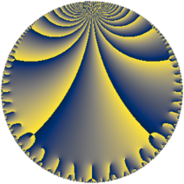# Properties

 Label 76.7.bLevel $76$ Weight $7$ Character orbit 76.b Rep. character $\chi_{76}(39,\cdot)$ Character field $\Q$ Dimension $54$ Newform subspaces $1$ Sturm bound $70$ Trace bound $0$

# Related objects

## Defining parameters

 Level: $$N$$ $$=$$ $$76 = 2^{2} \cdot 19$$ Weight: $$k$$ $$=$$ $$7$$ Character orbit: $$[\chi]$$ $$=$$ 76.b (of order $$2$$ and degree $$1$$) Character conductor: $$\operatorname{cond}(\chi)$$ $$=$$ $$4$$ Character field: $$\Q$$ Newform subspaces: $$1$$ Sturm bound: $$70$$ Trace bound: $$0$$

## Dimensions

The following table gives the dimensions of various subspaces of $$M_{7}(76, [\chi])$$.

Total New Old
Modular forms 62 54 8
Cusp forms 58 54 4
Eisenstein series 4 0 4

## Trace form

 $$54q - 10q^{2} + 90q^{4} - 44q^{5} - 510q^{6} + 440q^{8} - 13122q^{9} + O(q^{10})$$ $$54q - 10q^{2} + 90q^{4} - 44q^{5} - 510q^{6} + 440q^{8} - 13122q^{9} - 2088q^{10} - 2384q^{12} - 828q^{13} + 4116q^{14} - 7782q^{16} + 12220q^{17} + 15790q^{18} - 7000q^{20} - 16048q^{21} - 51864q^{22} + 19294q^{24} + 218346q^{25} - 18110q^{26} + 97926q^{28} - 101996q^{29} + 50076q^{30} - 235160q^{32} + 9072q^{33} - 51156q^{34} - 48028q^{36} - 63420q^{37} + 157260q^{40} - 135700q^{41} + 302290q^{42} + 137784q^{44} - 50348q^{45} - 177084q^{46} + 111320q^{48} - 1099338q^{49} + 485770q^{50} + 232980q^{52} + 892932q^{53} - 730010q^{54} - 298644q^{56} - 702534q^{58} + 1231628q^{60} + 323700q^{61} + 1573116q^{62} - 1065942q^{64} + 587512q^{65} - 74604q^{66} - 1235974q^{68} + 217376q^{69} + 1163796q^{70} - 1228320q^{72} - 739428q^{73} - 1852264q^{74} - 563144q^{77} - 2516852q^{78} - 1597436q^{80} + 4660886q^{81} - 919236q^{82} + 4928476q^{84} - 737904q^{85} - 1444124q^{86} + 2679960q^{88} - 3415940q^{89} + 4702636q^{90} - 3230670q^{92} - 5021584q^{93} + 1881960q^{94} + 1838870q^{96} + 3483660q^{97} - 3419858q^{98} + O(q^{100})$$

## Decomposition of $$S_{7}^{\mathrm{new}}(76, [\chi])$$ into newform subspaces

Label Dim. $$A$$ Field CM Traces $q$-expansion
$$a_2$$ $$a_3$$ $$a_5$$ $$a_7$$
76.7.b.a $$54$$ $$17.484$$ None $$-10$$ $$0$$ $$-44$$ $$0$$

## Decomposition of $$S_{7}^{\mathrm{old}}(76, [\chi])$$ into lower level spaces

$$S_{7}^{\mathrm{old}}(76, [\chi]) \cong$$ $$S_{7}^{\mathrm{new}}(4, [\chi])$$$$^{\oplus 2}$$